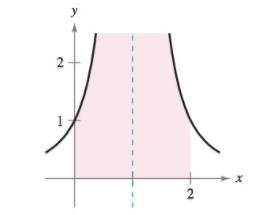Chapter 8.8, Problem 15E

Chapter
Section
Textbook Problem

Evaluating an Improper Integral In Exercises 13-16, explain why the integral is improper and determine whether it diverges or converges. Evaluate the integral if it converges. ∫ 0 2 1 ( x − 1 ) 2 d xTo determine

If the integral 021(x1)2dx is improper, will the integral converge and determine the value of the integral.

Explanation

Given:

The provided diagram is:

Explanation:

To check if the function is improper and determine the point of infinite discontinuity.

For this consider 1(x1)2= and find x:

1(x1)2=(x1)2=0x1=0x=1.

The point of discontinuity lies between upper limit and lower limit.

∴, it is concluded that given function is not continuous on interval [0,2].

Hence, given integral is improper.

Now since integral is discontinuous at x=1.

∴,

021(x1)2dx=011(x1)2dx+121(x1)2dx=limb1011(x1)2dx+limc1+121(x1)2dx

Integrate 1(x1)2:

limb1011(x1)2dx+limc1+121

Still sussing out bartleby?

Check out a sample textbook solution.

See a sample solution

The Solution to Your Study Problems

Bartleby provides explanations to thousands of textbook problems written by our experts, many with advanced degrees!

Get Started

Find more solutions based on key concepts